• 1、关于SVD分解 [U1,D1,V1] = svd(Ht,0); % Ht = U1 D1 V1' 如果Ht是10*5维，那么上行代码得到的结果为：U1 10*5, D1 5*5, V1 5*5; 否则[U1,D1,V1] = svd(Ht); % Ht = U1 D1 V1' 得到的结果为 U1 10*10, D1 10*5,...

1、关于SVD分解
[U1,D1,V1] = svd(Ht,0);     % Ht = U1 D1 V1'
如果Ht是10*5维，那么上行代码得到的结果为：U1 10*5, D1 5*5, V1 5*5;
否则[U1,D1,V1] = svd(Ht);     % Ht = U1 D1 V1' 得到的结果为 U1 10*10, D1 10*5, V1 5*5;

转载于:https://www.cnblogs.com/Jerry-PR/articles/5264435.html
展开全文• 最近用到LDA，因为不是数学方面出生，所以只管结果正确，不太追究具体的原理，在网上找到了一些别人的实现以及参考资料，自己实现了以下，但和OpenCV里面的LDA结果相差较大，后来经过参考多个版本以及理论知识，...
LDA 中文名称线性判别分析
最近用到LDA，因为不是数学方面出生，所以只管结果正确，不太追究具体的原理，在网上找到了一些别人的实现以及参考资料，自己实现了以下，但和OpenCV里面的LDA结果相差较大，后来经过参考多个版本以及理论知识，最终实现版本如下，与OpenCV的LDA结果一致且与LDA的公式相符：

function [coeff, latent]= myLDA(data, labels)
% Make sure labels are nice
[classes, bar, labels] = unique(labels);
nc = length(classes);

dataMean = mean(data, 1);
Sb = 0;%类间
for i = 1:nc
cur_X = data(labels == i,:);
meanTemp = mean(cur_X, 1);
Sb = Sb + size(cur_X,1)*(meanTemp'-dataMean')*(meanTemp'-dataMean')';
end

Sw = 0;%类内
for i = 1:nc
cur_X = data(labels == i,:);
meanTemp = mean(cur_X, 1);
for j = 1:size(cur_X,1)
dataTemp = cur_X(j, :);
Sw =  Sw + (meanTemp' - dataTemp')*(meanTemp' - dataTemp')';
end
end
Sb(isnan(Sb)) = 0; Sw(isnan(Sw)) = 0;
Sb(isinf(Sb)) = 0; Sw(isinf(Sw)) = 0;

[coeff, latent] = eig(Sw\Sb);
% Sort eigenvalues and eigenvectors in descending order
latent(isnan(latent)) = 0;
[latent, ind] = sort(diag(latent), 'descend');
[classes, ~, ~] = unique(labels);
no_dims = length(classes) - 1;
coeff = coeff(:,ind(1:min([no_dims size(coeff, 2)])));
end
测试数据1

data1 =

2.9500    6.6300
2.5300    7.7900
3.5700    5.6500
3.1600    5.4700<pre name="code" class="html">    2.5800    4.4600
2.1600    6.2200
3.2700    3.5200</pre><pre name="code" class="html"><pre name="code" class="html">label1 =
     0
0
0
0
1
1
1

结果如下：[因为LDA主要用特征向量，故此处只给出特征向量的结果，特征值在不同的实现当中会各有不同]

coeff =

-0.9231
-0.3846
测试数据2

data2 =

0     1
0     2
2     4
8     0
8     2
9     4<pre name="code" class="html">label2 =

0
0
0
1
1
1

结果如下：

coeff =

0.9204
-0.3909

另外在matlab里面有一个专门做数据降维的工具箱drtoobox，里面有实现LDA，但感觉它的计算结果不是我想要的那种，但如果修改了当中的一些代码，计算结果就会与我的一致，主要是最后计算特征值和特征向量，在drtoolbox当中为：

[M, lambda] = eig(Sb, Sw);改成以下则特征向量与我的一致：

[M, lambda] = eig(Sw\Sb);理论上两种计算应该都可以，但根据LDA的具体公式，个人更倾向于第二种方式。
另外由于matlab当中目前实现了PCA，会与drtoolbox当中的pca函数名冲突，在使用这个toolbox的时候需要修改其函数名，并修改compute_mapping里面用到pca函数的地方。
此处给出drtoolbox的介绍和官网：
  数据降维工具箱drtoolbox
 官网： Matlab Toolbox for Dimensionality Reduction

参考文章：
 OpenCV LDA(Linnear Discriminant analysis)类的使用---OpenCV LDA示例
 线性判别分析(Linear Discriminant Analysis, LDA）算法分析
 《模式分类》 机械工业出版社P96 - P101

展开全文• 今天小编为大家讲解数据预处理中的LDA线性判别分析。线性判别分析(Linear Discriminate Analysis,LDA)是一种特征抽取的技术，用以提高数据分析过程中的计算效率。我们在主成分分析(Principal Component Analysis,PCA...
今天小编为大家讲解数据预处理中的LDA线性判别分析。线性判别分析(Linear Discriminate Analysis,LDA)是一种特征抽取的技术，用以提高数据分析过程中的计算效率。我们在主成分分析(Principal Component Analysis,PCA)中将数据点进行降维时，考虑的是将数据整体向某一方向投影。但数据内部有类别标签时，PCA可能就显得不那么出色了。如下图。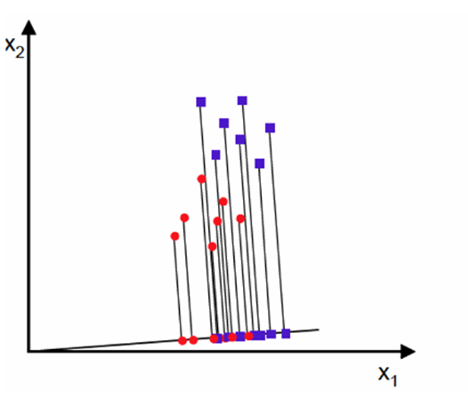虽然我们也可以看出数据在该投影方向上的密度划分，但数据类别(图中红点、蓝点)已经交织在一起，图像整体感觉很乱。我们换一种投影方式，如下图，这回图像能表达的信息明显清晰多了，红点、蓝点也有效分开。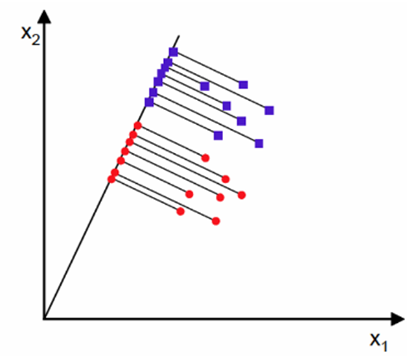该投影方向并不追求所有数据点垂直到向量的欧式距离之和最短，而是提出如下两个参数来确定最终方向：类间散度和类内散度。投影过后的类间散度和类内散度如下：有了上述两个重要参数，LDA的好坏程度就可以用J表示(Fisher线性判别)：公式分子为类间散度，越大越好。分母为类内散度，越小越好。定下来求解目标，数学推导过程如下(可不看)：μi为每类中心。上面带一个~的表示投影过后的点。上式SB就是类间散度，between-classscatterSW为类内散度，within-classscatter结合SB，SW式子转化成相对规整的形式J(W)对W求导等于0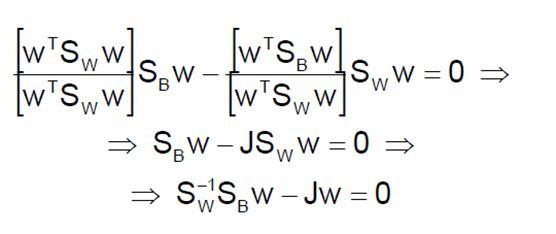得到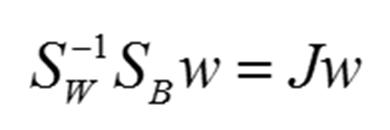公式中，J为矩阵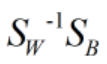的特征值。绕了一圈，LDA的求解又转换为求矩阵特征值的问题。以具有两类标签的数据为例，我们有如下数据：红叉和蓝叉。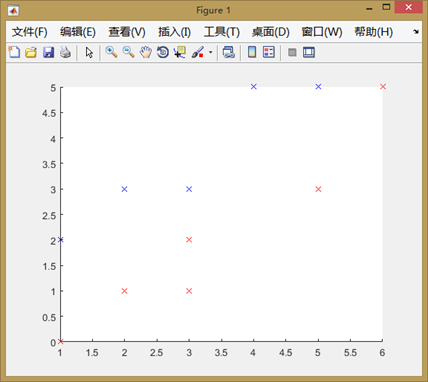计算，并求其特征值，和PCA问题一样，最大特征值对应的特征向量为求解向量方向。经matlab计算我们得到解w=(0.6656,-0.7463)。投影后图像如图(数据投影后为圈)：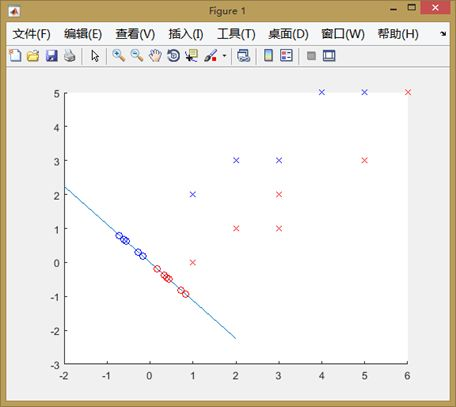我们看到，数据被降维，且被相对完好的分为两类。如果数据标签数量为3呢？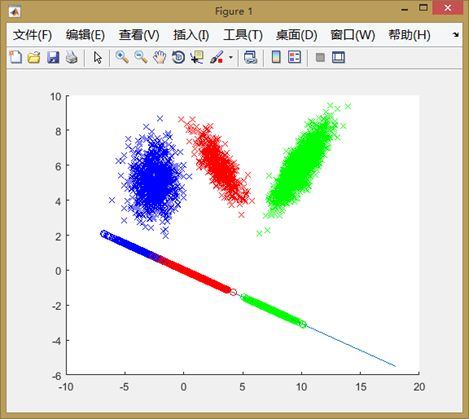我们看到，经投影后，数据在保证类内聚合度很高的情况下，类之间的耦合度也被降到最低。但是投影后数据点之间也难免会存在重叠的情况。这也说明，LDA在进行数据降维时也并不能做到完美，面对有些复杂数据降维效果也不是很优秀。但在很多领域，信息数据仅仅通过线性分析就可以找出其中规律，解决这些实际问题时LDA具有十分重要的意义。
展开全文• Matlab关于LDA人脸识别-lda.m 请问谁有一些关于 LDA 人脸识别的源程序！谢谢大家了！
• matlab编写程序，最终实现LDA分析，获得相应数据的结果。程序
• 各位大神们，我最近用MATLAB实现LDA，但是它的输入数据模式是id:count,即输入的是每个词在文档中出现的次数，而我现在有个现成的文档-词语概率矩阵，把这个当作输入，程序运行不起来，因为手头的这个程序是在词频的...
• % This code is LDA base face recoginition programme. It reads nots(here 6) % facse from ORL database and the rest (nump-nots) are used as test. % LDA_Performance shows the recognition performance. % ...
• lda implemented by matlab
• 是基于用MATLAB编程的LDA程序，程序运行完美，训练集设置较为巧妙，方便学习者自己添加数据，而且注释非常详细，不仅注释了每步的作用，而且对出现的函数也做了介绍
•线性判别分析
• LDA人脸识别matlab程序代码！ LDA人脸识别matlab程序代码！ LDA人脸识别matlab程序代码！ LDA人脸识别matlab程序代码！
• LDA算法对于两类问题的LDA(Matlab实现)1 function [ W] = FisherLDA(w1,w2)2 %W最大特征值对应的特征向量3 %w1 第一类样本4 %w2 第二类样本56 %第一步：计算样本均值向量7 m1=mean(w1);%第一类样本均值8 m2=mean(w2)...
LDA算法对于两类问题的LDA(Matlab实现)1 function [ W] = FisherLDA(w1,w2)2 %W最大特征值对应的特征向量3 %w1 第一类样本4 %w2 第二类样本56 %第一步：计算样本均值向量7 m1=mean(w1);%第一类样本均值8 m2=mean(w2);%第二类样本均值9 m=mean([w1;w2]);%总样本均值1011 %第二步：计算类内离散度矩阵Sw12 n1=size(w1,1);%第一类样本数13 n2=size(w2,1);%第二类样本数14 %求第一类样本的散列矩阵s115 s1=0;16 for i=1:n117 s1=s1+(w1(i,:)-m1)‘*(w1(i,:)-m1);18 end19 %求第二类样本的散列矩阵s220 s2=0;21 for i=1:n222 s2=s2+(w2(i,:)-m2)‘*(w2(i,:)-m2);23 end24 Sw=(n1*s1+n2*s2)/(n1+n2);25 %第三步：计算类间离散度矩阵Sb26 Sb=(n1*(m-m1)‘*(m-m1)+n2*(m-m2)‘*(m-m2))/(n1+n2);27 %第四步：求最大特征值和特征向量28 %[V,D]=eig(inv(Sw)*Sb);%特征向量V，特征值D29 A = repmat(0.1,[1,size(Sw,1)]);30 B = diag(A);31 [V,D]=eig(inv(Sw + B)*Sb);32 [a,b]=max(max(D));33 W=V(:,b);%最大特征值对应的特征向量34 end测试：cls1_data=[2.95 6.63;2.53 7.79;3.57 5.65;3.16 5.47];cls2_data=[2.58 4.46;2.16 6.22;3.27 3.52];%样本投影前plot(cls1_data(:,1),cls1_data(:,2),‘.r‘);hold on;plot(cls2_data(:,1),cls2_data(:,2),‘*b‘);hold on;W=FisherLDA(cls1_data,cls2_data);%样本投影后new1=cls1_data*W;new2=cls2_data*W;k=W(2)/W(1);plot([0,6],[0,6*k],‘-k‘);axis([2 6 0 11]);hold on;%画出样本投影到子空间点for i=1:4temp=cls1_data(i,:);newx=(temp(1)+k*temp(2))/(k*k+1);newy=k*newx;plot(newx,newy,‘*r‘);end;for i=1:3temp=cls2_data(i,:);newx=(temp(1)+k*temp(2))/(k*k+1);newy=k*newx;plot(newx,newy,‘ob‘);end;结果：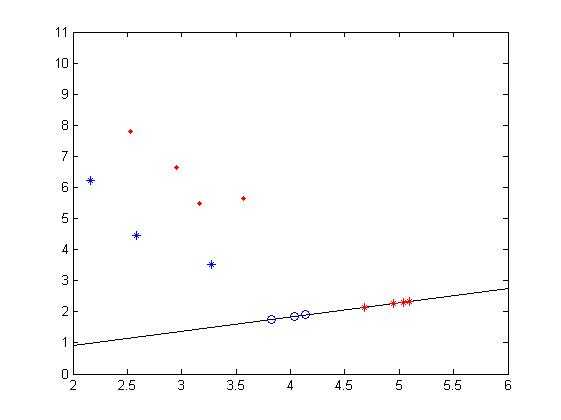原文：http://www.cnblogs.com/simon-c/p/4961942.html
展开全文• 本文使用LDA作为分类器在matlab下做实验。其中投影转换矩阵W按照LDA的经典理论生成，如下的LDA函数，并返回各个类的投影后的(ｋ－１)维的类均值。LDA.m代码如下：按 Ctrl+C 复制代码function [W,centers]=LDA(Input,...
• 代码如下 clear all;close all;clc; x=[0.697,0.774,0.634,0.608,0.556,0.403,0.481,0.437,0.666,0.243,0.245,0.343,0.639,0.657,0.360,0.593,0.719]; y=[0.460,0.376,0.264,0.318,0.215,0.237,0.149,0.211,0.091,0....
• blei提出的潜在狄利克雷算法的MATLAB实现
• LDA（Linear discriminate analysis） 分类器 。写成了MATLAB函数，效果还不错。
• ## LDAmatlab code

千次阅读 2014-02-19 13:39:29
% prophet Mohammed said [ALLAH will help any one helped his/her brother/sister] PBUH %This code to apply LDA (Linear Discriminant Analysis) % for any information please send to engalaatharwat@hotmail
• matlab中编写的Latent Dirichlet Allocation源码
• 主题模型（Topic Model）LDA详解及其Matlab代码
• 本文约2500字，含数学公式较多，预计阅读时间15分钟，阅读难度中等...全文分成如下四个部分：PCA 回顾线性判别分析(LDA)多类别的线性判别分析使用LDA的限制一 PCA回顾介绍LDA之前，简单的回顾一下之前讲到的PCA。PCA...
• 老外写的matlab写的LDA程序，初学者好用！
• ## LDA，matlab实现

热门讨论 2012-07-16 14:30:19
LDA（ Linear Discriminant Analysis），很不错
• 1 Linear Discriminant Analysis 相较于FLD（Fisher Linear Decriminant），LDA假设:1.样本数据服从正态分布，2.各类得协方差相等。虽然这些在实际中不一定满足，但是LDA被证明是非常有效的降维方法，其线性模型对于...
• 实用文案 LDA 人脸识别的 matlab 程序 以下是 LDA 的 m 文件函数 你稍稍改改就能用了 function [eigvector, eigvalue, elapse] = LDA(gnd,options,data) % LDA: Linear Discriminant Analysis % % [eigvector, ...
• 用于铜浮选工况识别的，多类训练集的线性判别分析源代码（matlab线性判别分析
• ## LDA算法(MATLAB实现)

热门讨论 2012-11-06 16:02:33...

# matlabldamatlab 订阅